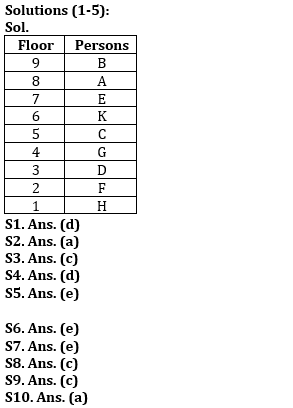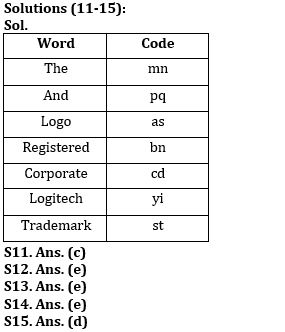Latest Banking jobs   »

# Reasoning Ability Quiz For SBI Clerk Prelims 2022- 15th May

Directions (1-5): Study the information carefully and answer the question given below.
Nine persons live in a building on 9 different floors in such a way that ground floor is numbered as 1 and top floor is numbered as 9. E lives on an odd number floor above the floor number 5. There are two persons live between E and G. B lives on odd number floor but not immediate above and immediate below G. There are as many persons live between G and F as live between G and K. F lives below G. H live on ground floor. There is one person lives between C and E. D does not live on an even number floor. A lives on even numbered floor but above on 2nd floor.

Q1. Which of the following is true with respect to A as per the given arrangement?
(a) Only three persons live between A and H
(b) Only three persons live above A.
(c) Only one person lives between A and G.
(d) A lives on even numbered floor.
(e) None of these.

Q2. On which of the following floor number does D live?
(a) Third
(b) First
(c) Second
(d) Fifth
(e) Seventh

Q3. Who lives on the floor immediately below C?
(a) F
(b) A
(c) G
(d) H
(e) E

Q4. How many persons live between the floors on which H and G live?
(a) Three
(b) More than three
(c) None
(d) Two
(e) One

Q5. Who lives on the floor numbered 5?
(a) F
(b) H
(c) G
(d) B
(e) None of these

Directions (6-10): Following questions are based on the five three-digit numbers given below.

947 376 694 739 863

Q6. If all the digits in each of the numbers are arranged in increasing order within the number, then, which of the following number will become the lowest in the new arrangement of numbers?
(a) 947
(b) 863
(c) 739
(d) 694
(e) 376

Q7. If all the numbers are arranged in ascending order from left to right then, which of the following will be the sum of all the three digits of the number which is 2nd from the right in the new arrangement?
(a) 18
(b) 19
(c) 15
(d) 16
(e) None of these

Q8. What will be the difference when third digit of the 3rd lowest number is multiplied with the second digit of the highest number and third digit of the 2nd highest number is multiplied with the second digit of the lowest number?
(a) 21
(b) 20
(c) 15
(d)16
(e) None of these

Q9. If the positions of the second and the third digits of each of the numbers are interchanged then, how many even numbers will be formed?
(a) None
(b) One
(c) Two
(d) Three
(e) Four

Q10. If one is added to the second digit of each of the numbers and one is subtracted from the third digit of each number then, how many numbers thus formed will be divisible by three in new arrangement?
(a) None
(b) One
(c) Two
(d) Three
(e) Four

Directions (11-15): Study the following information carefully and answer the given questions.
In a certain code language,
‘Logitech And the Logo’ is coded as ‘yi pq mn as’
‘Registered Trademark and the’ is coded as ‘mn bn st pq ‘
‘Registered Corporate the Logo’ is coded as ‘cd as mn bn’

Q11. Which of the following is the code for ‘and’?
(a) st
(b) bn
(c) pq
(d) mn
(e) None of these

Q12. Which of the following word is coded as ‘mn’?
(a) Corporate
(b) Logo
(d) Registered
(e) None of these

Q13. What may be the code of ‘Corporate society’?
(a) st pq
(b) cd mn
(c) pq bn
(d) mn pq
(e) cd qw

Q14. Which of the following code is coded for ‘logitech’?
(a) mn
(b) pq
(c) bn
(d) cd
(e) None of these

Q15. What will be the code of ‘the Logo’?
(a) st pq
(b) cd as
(c) cd pq
(d) mn as
(e) st bn

Solutions#### Congratulations!